# Diseño Óptimo De Tuberías en Serie

Este artículo presenta un enfoque que combina los conceptos de uso de energía y la Programación Lineal Entera (ILP) para establecer de cerca soluciones óptimas para el diseño óptimo de tuberías en sistemas de la serie en un tiempo reducido. La metodología propuesta permite predefinir la cabeza en cada nodo basado en criterios conocidos desarrollados por investigaciones anteriores en el diseño óptimo de redes cerradas con demandas guiadas. Una vez que las cabezas están disponibles, las demandas se calculan con la función de demanda de presión y entonces el problema se resuelve como impulsado por la demanda con la ILP. Teniendo en cuenta que el diseño resultante puede ser inviable debido a los probables cambios en las cabezas de los nodos y por lo tanto en los flujos de la demanda, se necesitan varias iteraciones de la metodología que explora el espacio de la asignación de cabeza de una manera inteligente. La metodología se prueba para diferentes escenarios que muestran las ventajas de este enfoque.

/var/www/pavco.com.co/public/site/pdftohtml/e43c839b41d9ae310c1dd0536f65d74c/index-html.htmlOptimal design of pipes in series with pressure driven

demands

Páez, D.

1

, Hernandez, D.

2

and Saldarriaga, J.

3

ABSTRACT

This paper presents an approach that combines concepts of energy use and the ILP to
found  near  optimal  solutions  for  the  optimal  design  of  pipes  in  series  systems  in  a
reduced  amount  of  time.  The  proposed  methodology  predefines  the  head  in  each
node  based  on  known  criteria  developed  by  past  research  on  optimal  design  of
looped  demand-driven  networks.  Once  the  heads  are  available,  the  demands  are
calculated  with  the  demand-pressure  function  and  then  the  problem  is  solved  as
demand-driven  with  ILP.  Taking  into  account  that  the  resulting  design  can  be
unfeasible because of the probable changes in the nodes’ heads and therefore in the
demand flows, there are needed various iterations of the methodology that explores
the  head  assignation  space  in  an  intelligent  way.  The  methodology  is  tested  for
different scenarios showing the advantages of this approach.

Keywords:

Pipe in series, pressure driven demands, Optimal Hydraulic Gradient Line (OHGL),
Integer Linear Programming (ILP)

1

Professor,  Civil  and  Environmental  Engineering  Department,  Universidad  de  los  Andes.

Researcher,  Water  Distribution  and  Sewer  Systems  Research  Center  –  CIACUA.  Email:

da.paez27@uniandes.edu.co

2

Researcher,  Water  Distribution  and  Sewer  Systems  Research  Center  –  CIACUA.  Civil  and

Environmental

Engineering

Department,

de

los

Andes.

Email::

da.hernandez39@uniandes.edu.co;

3

Professor, Civil and Environmental Engineering Department, Universidad  de los Andes. Director,

Water

Distribution

and

Sewer

Systems

Research

Center

–

CIACUA.

Email:

jsaldarr@uniandes.edu.co

/var/www/pavco.com.co/public/site/pdftohtml/e43c839b41d9ae310c1dd0536f65d74c/index-html.html1.

INTRODUCTION

A pipe in series is a type of water distribution system (WDS) in which there is one
reservoir, a set of pipes connected in a lineal way and a set of demand nodes  placed
on  the  pipes’  junctions.  The  system  is  usually  called  demand-driven  whenever  the
demand  on  the  nodes  is  independent  from  the  network‘s  hydraulic  behavior.
Likewise,  a  pressure-driven  model  is  one  in  which  the  demand  on  each  node  is  a
function of the systems pressure.

The optimal designing of WDSs consists in choosing the diameter of each pipe in the
system  ensuring  that  the  pressure  nodes  is  greater  than  or  equal  to  a  minimum
allowable  limit,  seeking  to  minimize  the  system’s  construction  cost.  Several
methodologies  have  been  used  to  design  demand-driven  models.  Most  of  those
methodologies  consist  in  heuristics  that  mimic  natural  and  physical  phenomena  to
explore  the  solution  space  e.g  Genetic  Algorithms  (Savic  &  Waters,  1997;  Wu  &
Simpson,  2001;  Reca  &  Martínez,  2006),  Simulated  Annealing  (Cunha  &  Sousa,
1999;  Reca  et  al.,  2007),  Harmony  Search  (Geem,  2002;  Gemm,  2009)  and  Ant
Colony  (Zecchin  et  al.,  2006;  Ostfled  & Tubaltzev,  2008),  among  others;  but  some
researchers  as  Ipai  Wu  in  1975  and  Ochoa  and  Saldarriaga  in  2009  have  proposed
methodologies based on hydraulic/energy concepts as Optimal Pressure  Grade  Line
and Optimal Power Use Surface.

Meanwhile,  for  pressure-driven  models  there  have  been  proposed  and  tested  less
number  of  methodologies  like  Genetic  Algorithms  (Farmani  et  al.,  2007),  Fuzzy
Linear  Programing  (Spiliotis  and  Tsakiris,  2007)  and  Recursive  Design  (González-
Cebollada et al., 2011); most of which are applied to design irrigation networks with
emitters at their nodes.

This paper presents an approach that combines the mentioned concepts of energy use
and Integer Linear Programming (ILP) to found near optimal solutions in a reduced
amount  of  time  for  pipes  in  series  systems  with  pressure-driven  demands.  This
research is considered a first step for further methodologies that attempt to solve the
WDS  optimal  design  problem  for  pressure  driven  demands  in  more  complex
networks and based on hydraulics and not in heuristics. It can be especially useful for
fire  water  networks  design,  WDSs  design  considering  leakage,  residential and  non-
residential plumbing systems design among others.

2.

PROBLEM FORMULATION

This study deals with pressure-driven demand models with a pipes in series topology.
The  optimal  design  can  be  defined  as:  Given  a  layout,  lengths  of  each  pipe,
topography,  connection  between  pipes  and  nodes  and  the  minimum  pressure
requirement,  find  the  diameter  combination  that  implies  the  minimum  construction
cost.  This  combination  must  obey  the  mass  and  energy  conservation  principles  and
the  minimum  pressure  requirement  on  each  node  (in  this  study  there  are  not

/var/www/pavco.com.co/public/site/pdftohtml/e43c839b41d9ae310c1dd0536f65d74c/index-html.htmlconsidered  other  kind  of  constraints  like  minimum  and  maximum  velocities).
Mathematically, the problem can be expressed as:



where

is pipe in series construction cost and is calculated as:

∑



where

is the number of pipes in the series;

is  the  length  of  pipe

;

is  the

diameter of pipe

; and   and   are regression parameters for the pipe unitary costs

as a function of the diameter. Problem constraints are:

Mass conservation:

(

)



where

is the total flow rate at pipe

,

is the base demand at node

, and

is

the  flow  of  the  emitter  at  node

and  it depends of the pressure on  that node as is

shown in Equation 4.



where

is the pressure head in node

, and

and

are coefficients that describe

the emitter characteristics.

Energy conservation:



where

is the total head in node

,

is the total head at the reservoir,

is the

friction loss in pipe

;

is the minor loss in pipe

and    is the number of nodes.

For this study friction losses are calculated with Darcy-Weisbach equation.

Minimum pressure in demand nodes:



where

is  the  minimum  head  required in node

which corresponds with the

minimum allowable pressure.

Pipe diameters can only  take discrete values belonging to commercial diameters  set



/var/www/pavco.com.co/public/site/pdftohtml/e43c839b41d9ae310c1dd0536f65d74c/index-html.html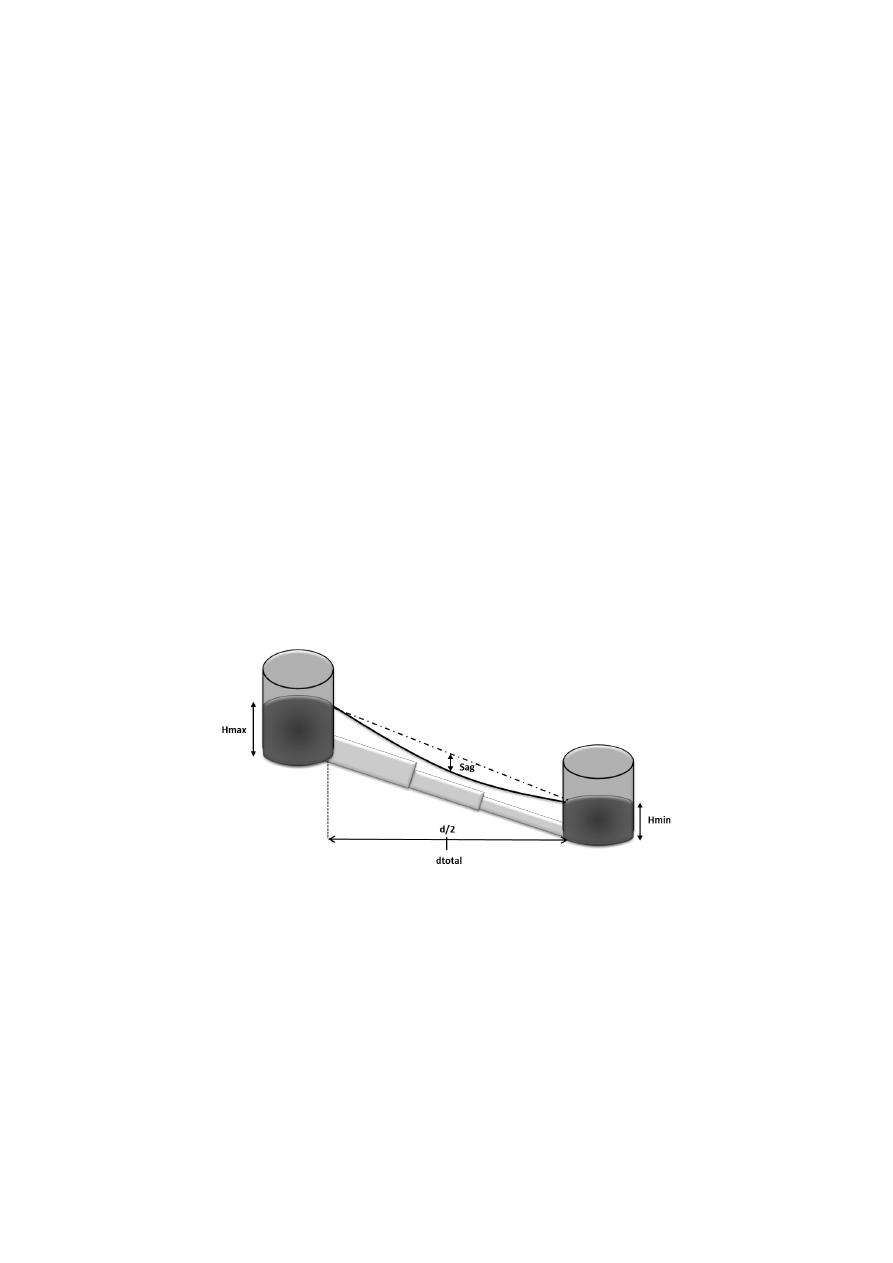It should be noticed that the flow in each node is not known before the design as they
depend of the pressure on each downstream node, and for that reason IPL cannot be
used directly to find the global optimum of the problem.

3.

OPTIMUM HYDRAULIC GRADE LINE FOR A PIPE IN SERIES

As well as I-pai Wu (1975) and later Ochoa and Saldarriaga (2009) established, the
minimum cost design usually develops a parabolic hydraulic gradient line (HGL). In
order  to  establish  the  behavior  of  the  quadratic  equation  of  the  hydraulic  gradient,
there must be known three points that describes the parabolic function. In the case of
the hydraulic gradient, the three points are:

Hmax:  is  the  available  head  for  the  entire  network  and  as  it  is  the  head  at  the
reservoir, it is placed at abscissa

.

Hmin: is the  minimum  head for the critical node  which is either the final node or a
node that will have a total head closer to the minimum because of its elevation. As it
defines the final node, it is placed at abscissa

.

Hsag:  corresponds  to  the  head  in  the  point  of  maximum  curvature  in  the  hydraulic
gradient line. This point is defined by the Sag which is a percentage of the difference
between Hmax and Hmin line and it will determine the Hsag as shown in Figure 1. It
is always placed at abscissa

.

Figure 1. HGL goal, based on three known points.

As shown in Figure 1 it can be seen that there is a straight line corresponding to the
case when the hydraulic gradient line is linear. When the Sag is 0 the gradient will be
equal to the straight line, but when the sag is different to 0 the head in the middle of
the  pipe  system  will  be  equal  to  the  head  in  the  middle  point  for  the  straight  line
minus the Sag multiplied by the available head in the system.

This  means  that  the  objective  hydraulic  gradient  line  could  be  found  by  this
expression:

/var/www/pavco.com.co/public/site/pdftohtml/e43c839b41d9ae310c1dd0536f65d74c/index-html.html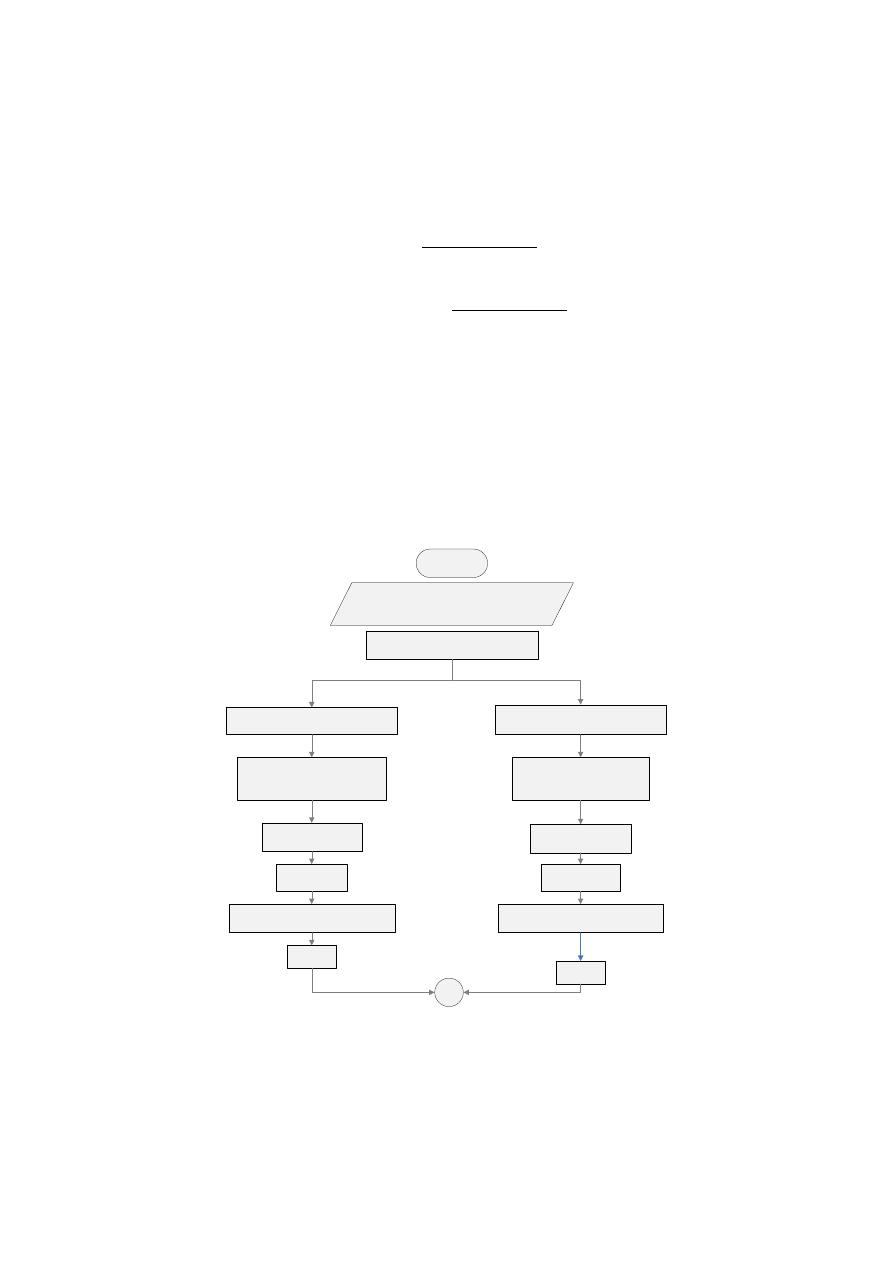where:

is the objective

placed at a distance   from the reservoir; S is

the selected sag and

and

are the heads at the mentioned points.

4.

METODOLOGY

The proposed methodology is show in Diagram 1 and explained above:

Predifine HGL for Sag 0 and 0.25 using

Equation

Calculate flow on each node  for sag 0 HGL

Assign the flow obtained as the total

base demand on each node

Calculate flow on each node  for sag 0.25 HGL

Assign the flow obtained as the total

base demand on each node

Design the system ussing

Integer Linear

Programming

Design is obtained

S(0) = D1

Design is obtained

S(0.25) = D2

Run D1 hydraulics with pressure driven

demands in each node and calculate the

pressure and real flow on each node.

Run D2 hydraulics with pressure driven

demands in each node and calculate the

pressure and real flow on each node.

Print:

FlowP1_i,

Print:

FlowP2_i,

Design the system ussing

Integer Linear

Programming

A

START

(Hmax), Minimum Pressure (Pmin),

diameters, Ks, Km.

Diagram 1. Methodology.

/var/www/pavco.com.co/public/site/pdftohtml/e43c839b41d9ae310c1dd0536f65d74c/index-html.html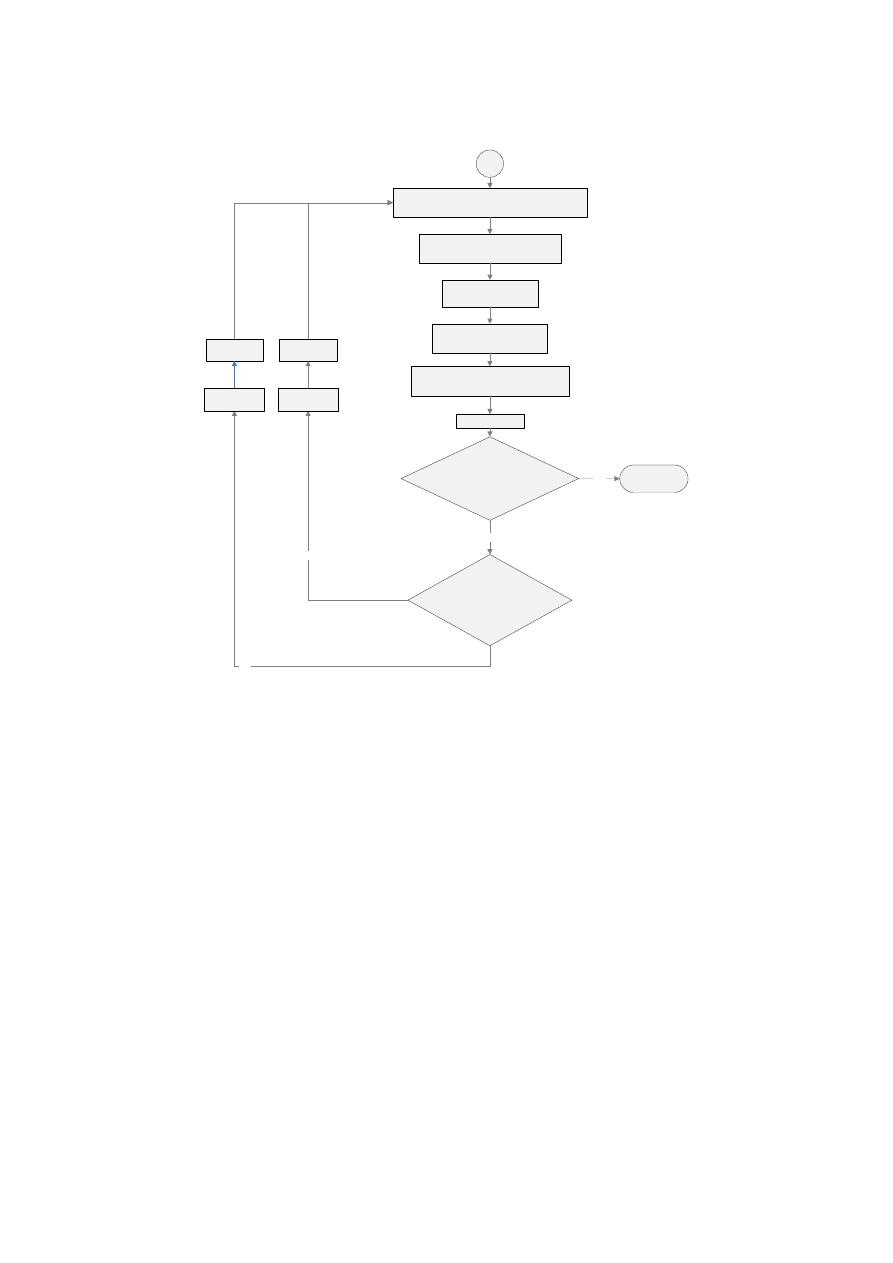FlowMj_i = (FlowP1_i + FlowP2_i )/2

Assign FlowMj_i on each node i

Design the system ussing

Integer Linear

Programming

Run Dj hydraulics with pressure driven

demands in each node and calculate the

pressure and real flow on each node.

min are greater than Zero?

Print: Design_Average j = Dj

YES

FlowP1_i =

FlowPj_i

Print: FlowPj_i

FlowP2_i =

FlowPj_i

IF(

∑FlowE_ji - ∑FlowE_j-1i < ε  )

AND

NO

NO

Yes

D2 = Dj

D1 = Dj

A

END

Diagram 2. Methodology.

In order to calculate the HGL is important determine the sag that will be used. It can
be  proved  that  the  sag  have  a  validity  range  between  0  and  0.25.  When  design  is
based  on  the  HGL  with  a  0  sag  the  system  meets  the  minimum  pressure  restriction
but generates high constructive costs as it overestimates the emitter flows. Whereas
when is based on a 0.25 sag the design has low constructive cost but does not meet
the minimum pressure because of its subestimation of emitter flows.

Considering that behavior, the methodology looks for an average between those two
designs looking to accurately estimate the emitter flows and therefore the flow rate in
each pipe.

4.2 Designs with Integer Linear Programming

Once the flow rate in each pipe is supposed by using the results of the last step, the
optimum design of that system can be achived with the following ILP formulation:

/var/www/pavco.com.co/public/site/pdftohtml/e43c839b41d9ae310c1dd0536f65d74c/index-html.htmlDefine

as the set of nodes in the network,

as the set of available diameters and

as binary decision variables described by Equation :

{



Also define

as

auxiliary decision variables that represent the total head in the node

i

N. Then the objective function is:

∑ ∑ ∑



were

is the cost of assigning a diameter

in the pipe that goes from node

to the node      . Finally the constraints for the ILP problem are:

Constraints:

Constraint of minimum allowable pressure and its consequent total head

defined by Equation 6.

Constraint that ensures the conservation of energy for each pipe. The total

downstream the node       will be equal to the total head

in node

minus the total head losses produced in the pipe from       to

when a diameter       is assigned to that pipe:

∑

,          |



were

is the head in the downstream node,

node,

is the parameter of total head losses that occurs in pipe from node

to       when a diameter       is assigned, and         is a function
that returns a

when the pipe that goes from   to   acctually exists ad a

otherwise.

Constraint that ensures that only one diameter is assigned to each pipe:

∑

,          |



This  formulation  was  implemented  in  the  program  Xpress  IVE.  The  Xpress-
Optimizer  features  sophisticated,  robust  multi-threaded  algorithms  to  quickly  and
accurately solve linear problems (LP).

After this step the designer will have two different designs, the design obtained from
Sag 0 (D

1

) and the design obtain from Sag 0.25 (D

2

). It is important to mention that,

the design D

1

will be more expensive than the design D

2

; but on the other hand, the

design D

1

will be feasible hydraulically and D

2

probably won’t. This is verified in the

next step.

/var/www/pavco.com.co/public/site/pdftohtml/e43c839b41d9ae310c1dd0536f65d74c/index-html.html4.3 Hydraulic execution with pressure driven demands

As explained before, the designs D

1

and D

2

were obtained from constant demands, so

it  is  necessary  to  verify  their  hydraulic  behavior  when  they  are  modeled  with
pressure  driven  demands.  In  case that  the  design  D

2

results  in  a  feasible  design  the

algorithm ends and the final design will be D

2

, otherwise the process continue to next

step.

The  flows  for  each  node  calculated  with  the  hydraulic  execution  of  D

1

and  D

2

considering pressure-driven demands are stored. For D

1

the flow is Flow1

and for D

2

is Flow2

is the node ID.

4.4 Iteration process

1. Using the flows for each design (Flow1

i

and Flow2

i

) after hydraulic execution, a

new  estimation  of  the  flow  for  each  node  is  calculated  with  Equation  13  for  each
node

.



where

corresponds to the new flow in the node  for the next design called

Dm

(the middle design).

2. Assign

as a constant demand on each node.

3. Design the new middle system with LP using steps described at section 4.4.

4.  Verify  the  hydraulic  performance  for  design  D

m

total flow in each node

5.  Restore  D

or  D

2

:  The  designer  gets  to  this  step  due  to  the  unfeasibility  of  D

m

and/or  because  a  cheaper  design  is  expected  by  reducing  even  more  the  supposed
flow  for  each  node.  In  this  step  the  designer  has  to  observe  the  number  of  nodes
under the minimum pressure in D

m

In the presented conditional of this step, it can be observed that it starts a bisection
process.  After  replacing  D

m

in  D

2

or  D

1

,  the  designer  has  to  go  back  to  step  1  and

repeat  the  process  until  the  differences  between  the  flows  supposed  by  the  last  D

m

and the actual D

m

are negligible.

5.

RESULTS

The proposed methodology was tested  on 4 systems with a similar layout (15 pipes
in series) but with differences in topography and base demand on the nodes. Network
1, has no base demand and flat topography, Network 2 has a 240 Lps based demand

/var/www/pavco.com.co/public/site/pdftohtml/e43c839b41d9ae310c1dd0536f65d74c/index-html.html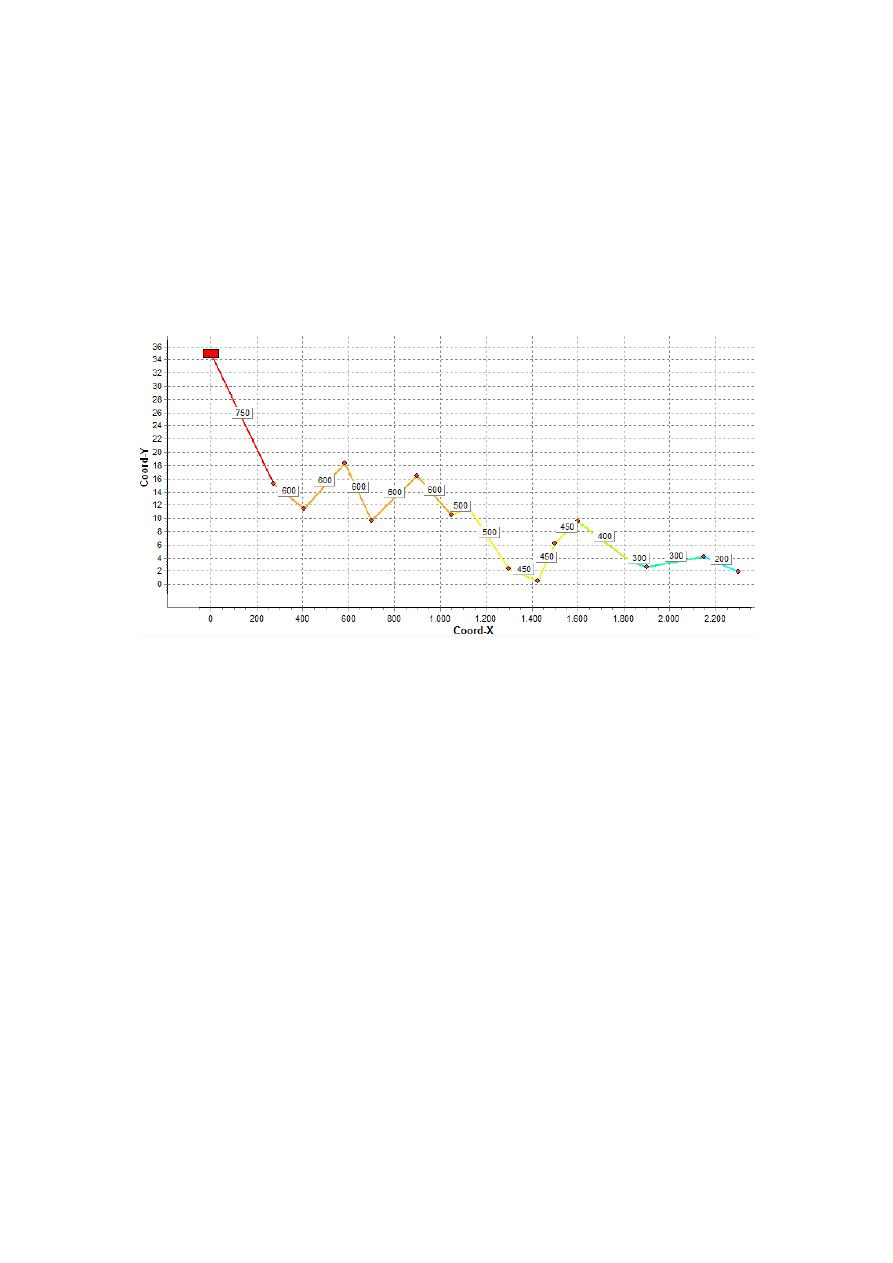and flat topography, Network 3 has no base demand and a topography presented on
Figure 2, and Network 4 has the same topography and a 240 Lps base demand. The
total  head  at  the  reservoir  is  35.0  m  and  the  minimum  allowable  pressure  for  the
nodes  is  10.0  m.  The  available  diameters  are  50,  75,  100,  150,  200,  250,  300,  350,
400, 450, 500, 600, 750, 800 and 1000 mm for networks 1 and 3 but considering the
base demand of networks 2 and 4, diameters of 1200, 1400 and 1500 were added to
the  list.  The  roughness  of  the  pipes  is

m  and  cost  parameters  were

and         . There were no minor losses considered on these networks.

Figure 2. Topography configuration for Network 3 and Network 4 and pipes’ lengths for the

four study cases.

The comparison was made with the SOGH methodology proposed by Ochoa (2009)
which is the methodology that presents the criterion of parabolic HGL to minimize
constructive costs. As the Sag mentioned above is a free parameter of the parabolic
equation, a design was made for different sag values.

On the other hand, the proposed methodology was implemented in REDES software
for  hydraulic  executions  developed  by  CIACUA  as  well  as  Xpress-IVE  for  ILP
problems’ solution. The results are presented on the following figures:

/var/www/pavco.com.co/public/site/pdftohtml/e43c839b41d9ae310c1dd0536f65d74c/index-html.html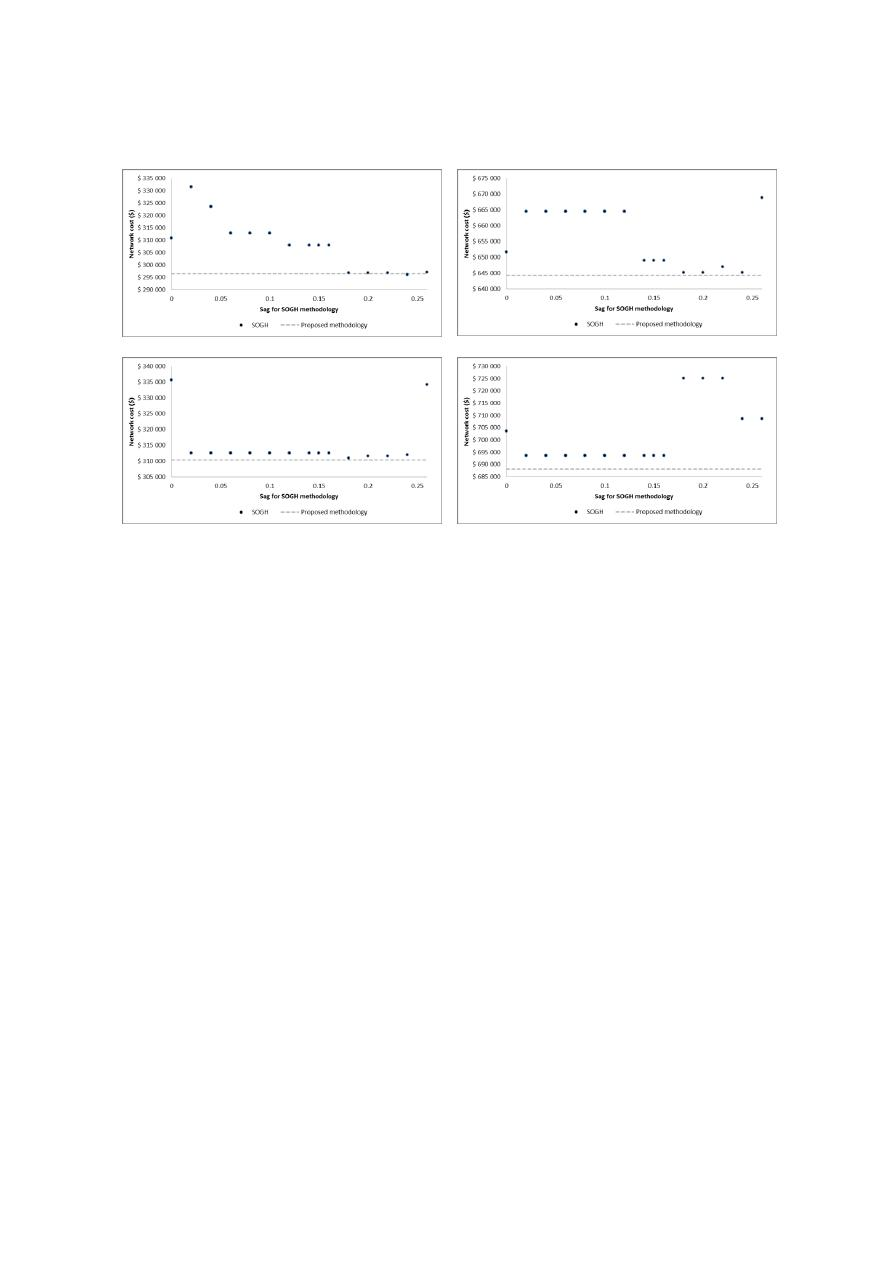a)

b)

c)

d)

Figure 3. Costs results for the four study cases. a) Network 1, b) Network 2, c) Network 3 and d)

Network 4.

It can be seen that the proposed methodology achieves designs with less constructive
costs than SOGH for three of the four study cases, and for the Network 1, a design
that costs 0.13% more than the better result found with SOGH. That means that the
ILP methodology is actually finding near optimal solutions in all the networks.

The  next  criterion  that  is  compared  is  the  computational  time  required  to  find  that
designs. For the Network 1 the best design found by SOGH (sag = 0.24) required 43
hydraulic  executions  of  the  system;  for  Network  2  the  best  design  can  be  achieved
with the sag values 0.18, 0.2 and 0.24 requiring 40 hydraulic executions; for Network
3  the  minimum  cost  sag  was  0.18  with  88  hydraulic  executions;  and  finally  the
Network 4 best design with SOGH required 71 hydraulic executions with a 0.02 sag
but  that  same  design  can  be  found  using  sags  between  0.02  and  0.16,  each  value
requiring different number of hydraulic executions.

It  should  be  noticed  that  the  previous  number  of  executions  required  by  SOGH
methodology  are  actually  the  executions  required  if  you  know  a  priori  the  optimal
sag,  but  it  is  a  difficult  task  as  it  depends  on  the  demand  distribution  among  the
system, and as it is pressure-driven it is not known before the design. Therefore the
SOGH methodology required actually 568 hydraulic executions for Network 1, 591
for Network 2, 1019 for Network 3 and 1345 for Network 4, which were used in 14
different designs for each network with sags varying from 0.02 to 0.26.

On the other hand the computational time spent by the ILP methodology is composed
by  the  time  assigning  the  HGL,  which  is  negligible,  the  time  defining  each  ILP
formulation,  which  requires  the  calculation  of  each

/var/www/pavco.com.co/public/site/pdftohtml/e43c839b41d9ae310c1dd0536f65d74c/index-html.htmloccurs in pipe from node

to       when a diameter       is assigned), the ILP

problems’ solving and the hydraulic executions required after each ILP formulation.

The

computing can be done by assigning  to the entire system the diameter

and then executing the hydraulics reading the head losses on the pipes, and repeating
that process for each available diameter. The ILP solving lasted less than 0.1 seconds
on a Intel Core i5 processor with 3.0 GB RAM Memory using Xpress-IVE software,
so it is also negligible when compared with the hydraulic executions.

Therefore  the  proposed  methodology  required  80  hydraulic  executions  for  the
Network  1,  but  75  of  those  were executions  with  constant  demand  on  the  nodes as
there  were  used  just  for  the  computing  of  the

and  only  5  executions  were

actually done with the system with pressure-driven demands. Considering the way in
which  the  pressure-driven  demand  models  are  executed  with  the  Gradient  Method
programmed  in  EPANET  (Rossman,  2000)  and  also  in  REDES  software,  the  75
hydraulic  executions  with  constant  demand  plus  the  5  executions  with  pressure-
driven  demands  are  barely  more  time  demanding  than  the  43  executions  with
pressure driven demand spent by SOGH.

In  the  case  of  Network  2,  the  ILP  methodology  required  36  hydraulic  executions
with constant demands and 2 executions with pressure-driven demands, resulting in
fewer  executions  than  the  best  design  accomplished  by  SOGH.  For  Network  3,  60
hydraulic  executions  with  constant  demands  and  4  executions  with  pressure-driven
demands  were  required.  Finally  for  Network  4  were  spent  54  hydraulic  executions
with constant demands and 3 executions with pressure-driven demands.

It  means  that  the  proposed  methodology  can  achieve  near  optimal  designs  in  a
reduced amount of time considering pressure-driven demands using hydraulic criteria
for the definition of the HGL and ILP for the consequent diameters selection.

6.

CONCLUSIONS

A design methodology that uses hydraulic criteria to predefine an objective hydraulic
grade line and Integer Linear Programming to design a pressure-driven system, was
presented and tested on four study cases with a pipes’ in series topology, showing its
benefits  in  terms  of  the  quality  of  the  solutions  (reduced  constructive  cost)  and  the
amount of computational time required (reduced number of hydraulic executions).

This  study  is  a  first  step  to  develop  further  methodologies  that  solves  the  WDS
optimal  design  problem  for  pressure  driven  demands  in more complex  networks. It
can  be  especially  useful  for  fire  water  networks  design,  WDSs  design  considering
leakage, residential and non-residential plumbing systems design among others.

/var/www/pavco.com.co/public/site/pdftohtml/e43c839b41d9ae310c1dd0536f65d74c/index-html.html7.

REFERENCES

Cunha,  M.  a.  (1999).  Water  distribution  network  design  optimization:  Simulated

annealing approach. J. Water Resour. Plan. Manage. , 215-221

Farmani, R, Abadia, R. and Savic, D. (2007) “Optimum Design and Management of

Pressurized Branched Irrigation Networks” J. Irrig. Drain Eng., 133(6), 528–
537.

Geem,  Z.  K.  (2002).  Harmony  search  optimization:  Application  to  pipe  network

design. Int. J. Model. Simulat. , 125-133.

Geem,  Z.  K.  (2009).  Particle-swarm  harmony  search  for  water  network  design.

Engineering Optimization, Vol.41, No.4, pp. 297-311.

González-Cebollada, C., Macarulla, B., and Sallán, D. (2011). ”Recursive Design of

Pressurized Branched Irrigation Networks.” J. Irrig. Drain Eng., 137(6), 375–
382.

Ochoa, S. (2009). Optimal design of water distribution systems based on the optimal

hydraulic  gradient  surface  concept.  MSc  Thesis,  dept.  of  Civil  and
Environmental  Engineering,  Universidad  de  los  Andes,  Bogotá,  Col.  (In
Spanish).

Ostfeld,  A.  and  Tubaltzev,  A.  (2008).  ”Ant  Colony  Optimization  for  Least-Cost

Design  and  Operation  of  Pumping  Water  Distribution  Systems.”  J.  Water
Resour. Plann. Manage., 134(2), 107–118.

Reca,  J.  and  Martínez,  J.  (2006).  Genetic  algorithms  for  the  design  of  looped

irrigation  water  distribution  networks.  Water  Resources  Research,  Vol.44,
W05416

Reca,  J.,  Martínez,  J.,  Gil,  C.  and  Baños,  R.  (2007).  Application  of  several  meta-

heuristic  techniques  to  the  optimization  of  real  looped  water  distribution
networks. Water Resources Management, Vol.22, No.10, pp. 1367-1379.

Saldarriaga,  J.  (2007).  “Hidráulica  de  Tuberías.  Abastecimiento  de  Agua,  Redes,

Riego”. Bogotá: Alfaomega.

Savic,  D.  and  Walters,  G.  (1997).  Genetic  algorithms  for  least  cost  design  of  water

distribution networks. J. Water Resour. Plan. Manage , 67-77.

Spiliotis,  M.  and  Tsakiris,  G.  (2007).  ”Minimum  Cost  Irrigation  Network  Design

Using  Interactive  Fuzzy  Integer Programming.” J. Irrig. Drain Eng., 133(3),
242–248.

Wu,  I.  (1975).  Design  of  drip  irrigation  main  lines.  Journal  of  Irrigation  and

Drainage Division, Vol.101, No.4, pp. 265-278.

Wu, Z. and Simpson, A. (2001). ”Competent Genetic-Evolutionary Optimization of

Water Distribution Systems.” J. Comput. Civ. Eng., 15(2), 89–101.

Zecchin,  A.,  Simpson,  A.,  Maier,  H.,  Leonard,  M.,  Roberts,  A.,  and  Berrisfors,  M.

(2006)  Application  of  two  ant  colony  optimization  algorithms  to  water
distribution  system  optimization.  Mathematical  and  Computer  Modeling
Vol.44, No. 5-6, pp. 451-468